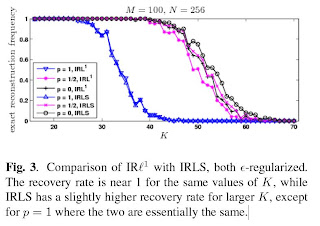Page Views on Nuit Blanche since July 2010

My papers on ArXiv:
Approximating Kernels at the speed of Light
&
Imaging with Nature

LightOn
LinkedIn (727)|| on CrunchBase || our Blog
(2452)
(3967)
(1333)||
Attendant references pages:
The Advanced Matrix Factorization Jungle Page ||

Paris Machine Learning
@Meetup.com (8016 members) || @Archives

Saturday, October 27, 2007

Compressed Sensing: Reweighted Lp through Least square (L2), Forget Europa, let's shoot for TitanIn a previous entry, I mentioned the impressive improvement of the L1 reconstruction capability for signals that were not so sparse. We also knew that an Lp (p less than 1) exact reconstruction of sparse signals via nonconvex minimization was also possible.

Based on the work of Bhaskar Rao and Kenneth Kreutz-Delgado , Rick Chartrand and Wotao Yin produced an astounding reconstruction technique in this article :

ITERATIVELY REWEIGHTED ALGORITHMS FOR COMPRESSIVE SENSING

The theory of compressive sensing has shown that sparse signals can be reconstructed exactly from remarkably few measurements. In , it was shown empirically that using Lp minimization with p less than 1 can do so with fewer measurements than with p = 1. In this paper we consider the use of iteratively reweighted algorithms for computing local minima of the nonconvex problem. In particular, a particular regularization strategy is found to greatly improve the ability of a reweighted least-squares algorithm to recover sparse signals, with exact recovery being observed for signals that are much less sparse than required by an unregularized version (such as FOCUSS, ). Improvements are also observed for the reweighted-l1 approach of .

Why is this is an important paper ? Because there is
• no need to use SDP programming, the scheme is very simple computationally. It can be written in ten lines of Matlab (Update: This algorithm was featured in this Monday Morning Algorithm)
• everybody in engineering knows least squares
• some of us now have a stab at the very large problems.

The major feature of this paper is showing that $\epsilon$ should probably be not that small in the first place. Let us hope that this heuristic will be proven in the future. In order to understand the graph above, one needs to know the acronyms:

• IRLS stands for Iteratively Reweighted or weighted Least Square (the algorithm of this paper)
• IRL1 stands for Iteratively Reweighted L1 (the case of p=0 is the same as the reweighted L1 algorithm in ).
K stands for the sparsity number of the signal, M is the number of measurements and N is the signal size.

Please note comparable numbers between IRL1/p=0 (linear programming algorithm in ) and IRLS/p=0 (least square algorithm in ).

Reference:
 ITERATIVELY REWEIGHTED ALGORITHMS FOR COMPRESSIVE SENSING, Rick Chartrand, Wotao Yin, ICASSP 2008

 An affine scaling methodology for best basis selection.
Rao, B.D.; Kreutz-Delgado, K. IEEE Transactions on Signal Processing, vol.47, (no.1), IEEE, Jan. 1999. p.187-200. [Rao1999.pdf]

 Enhancing sparsity by reweighted L1 minimization, Emmanuel Candès, Mike Wakin and Stephen Boyd